• 一、简介 clc clear all global box; global cargo;...%-------------------------------控制参数--------------------------- lambda = 0.5; % 重量利用率权重 T0 = 100; % 初始温度 T_End = 1;
一、算法原理
1、问题引入
之前我们了解过的算法大部分都是无约束优化问题，其算法有：黄金分割法，牛顿法，拟牛顿法，共轭梯度法，单纯性法等。但在实际工程问题中，大多数优化问题都属于有约束优化问题。惩罚函数法就可以将约束优化问题转化为无约束优化问题，从而使用无约束优化算法。
2、约束优化问题的分类
约束优化问题大致分为三类：等式约束、不等式约束、等式+不等式约束。
其数学模型为：
等式约束
min f(x),x\in R^n
s.t    hv(x)=0,v=1,2,…,p<n
不等式约束
min f(x),x\in R^n
s.t    gu(x)\leqslant 0,u=1,2,…,p<n
等式+不等式约束问题
min f(x),x\in R^n
s.t    hv(x)=0,v=1,2,…,p<n
s.t    gu(x)\leqslant 0,u=1,2,…,p<n
3、惩罚函数法定义
惩罚函数法（SUMT法）又称序列无约束极小化技术，将等式约束与不等式约束的条件，经过适当定义的复合函数加到原目标函数上构造了惩罚函数，从而取消了约束，转而求解一系列无约束优化问题。
按照惩罚函数再优化过程中的迭代点是否在约束条件的可行域内，又分为内点法、外点法和混合法
内点法：迭代点再约束条件的可行域之内，只用于不等式约束。
外点法：迭代点再约束条件的可行域之外，既用于不等式约束又可用于等式约束。
4、外点惩罚函数法
等式约束：
min f=x1.2+x2.2,x\in R^n
s.t    h1(x)=x1-2=0,h2(x)=x2+3=0
算法步骤
a、构造惩罚函数：F=f+M * { [ h1(x) ]^2 + [ h2(x) ]^2 } ，式中M为初始惩罚因子；
b、然后用无约束优化极值算法求解(牛顿法)；
c、   如果相邻两次惩罚函数无约束最优点之间的距离足够小【norm(x1-x0)<eps】，则收敛；
    否则放大惩罚因子M=C*M，式中C为 罚因子放大系数；

d、转步骤a继续迭代；
matlab代码
关于牛顿法的具体讲解，给出该博客网址https://blog.csdn.net/STM89C56/article/details/105643162
二、源代码
%% 外点惩罚函数法-等式约束
syms x1 x2
f=x1.^2+x2.^2;
hx=[x1-2;x2+3];%列

x0=[0;0];
M=0.01;
C=2;
eps=1e-6;
[x,result]=waidian_EQ(f,x0,hx,M,C,eps)

function [x,result]=waidian_EQ(f,x0,hx,M,C,eps)
% f 目标函数
% x0 初始值
% hx 约束函数
% M 初始罚因子
% C 罚因子放大系数
% eps 容差

%计算惩罚项
CF=sum(hx.^2);  %chengfa

while 1
F=matlabFunction(f+M*CF);%目标函数，使用之前的牛顿法，需要转换成句柄
x1=Min_Newton(F,x0,eps,100);
if norm(x1-x0)<eps
x=x1;
result=double(subs(f,symvar(f),x'));
break;
else
M=M*C;
x0=x1;
end
end
end
%牛顿法
function [X,result]=Min_Newton(f,x0,eps,n)
%f为目标函数
%x0为初始点
%eps为迭代精度
%n为迭代次数
TiDu=gradient(sym(f),symvar(sym(f)));% 计算出梯度表达式
Haisai=jacobian(TiDu,symvar(sym(f)));
Var_Tidu=symvar(TiDu);
Var_Haisai=symvar(Haisai);
Var_Num_Tidu=length(Var_Tidu);
Var_Num_Haisai=length(Var_Haisai);
TiDu=matlabFunction(TiDu);
flag = 0;
if Var_Num_Haisai == 0  %也就是说海塞矩阵是常数
Haisai=double((Haisai));
flag=1;
end
%求当前点梯度与海赛矩阵的逆
f_cal='f(';
TiDu_cal='TiDu(';
Haisai_cal='Haisai(';
for k=1:length(x0)
f_cal=[f_cal,'x0(',num2str(k),'),'];

for j=1: Var_Num_Tidu
if char(Var_Tidu(j)) == ['x',num2str(k)]
TiDu_cal=[TiDu_cal,'x0(',num2str(k),'),'];
end
end

for j=1:Var_Num_Haisai
if char(Var_Haisai(j)) == ['x',num2str(k)]
Haisai_cal=[Haisai_cal,'x0(',num2str(k),'),'];
end
end
end
Haisai_cal(end)=')';
TiDu_cal(end)=')';
f_cal(end)=')';
switch flag
case 0
Haisai=matlabFunction(Haisai);
dk='-eval(Haisai_cal)^(-1)*eval(TiDu_cal)';
case 1
dk='-Haisai^(-1)*eval(TiDu_cal)';
Haisai_cal='Haisai';
end
i=1;
while i < n
if abs(det(eval(Haisai_cal))) < 1e-6
disp('逆矩阵不存在！');
break;
end
x0=x0(:)+eval(dk);
if norm(eval(TiDu_cal)) < eps
X=x0;
result=eval(f_cal);
return;
end
i=i+1;
end
disp('无法收敛！');
X=[];
result=[];
end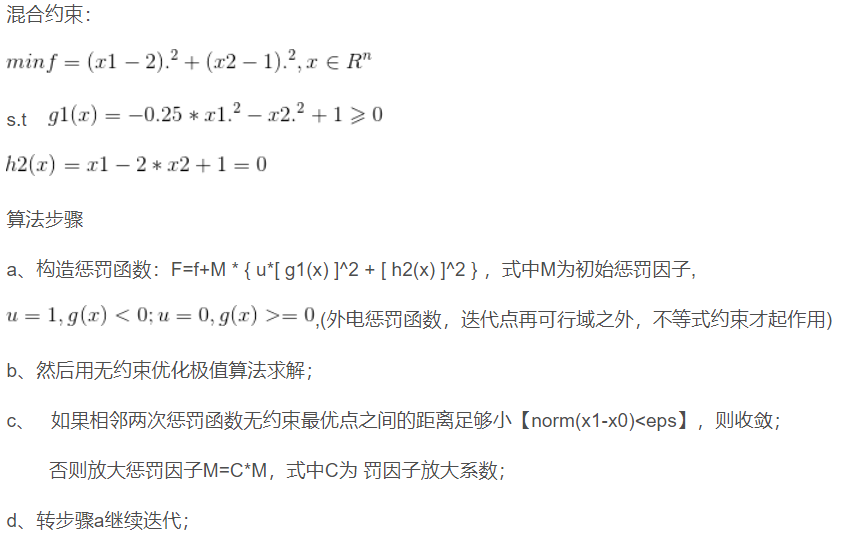%% 外点惩罚函数-混合约束
syms x1 x2
f=(x1-2)^2+(x2-1)^2;
g=[-0.25*x1^2-x2^2+1];%修改成大于等于形式
h=[x1-2*x2+1];

x0=[2 2];
M=0.01;
C=3;
eps=1e-6;
[x,result]=waidian_hunhe(f,g,h,x0,M,C,eps,100)

function [x,result]=waidian_hunhe(f,g,h,x0,M,C,eps,k)
% f 目标函数
% g 不等式约束函数矩阵
% h 等式约束函数矩阵
% x0 初始值
% M 初始惩罚因子
% C 罚因子放大倍数
% eps 退出容差
% 循环次数

CF=sum(h.^2);  %chengfa
n=1;
while n<k
%首先判断是不是在可行域内
gx=double(subs(g,symvar(g),x0));%计算当前点的约束函数值
index=find(gx<0);%寻找小于0的约束函数
F_NEQ=sum(g(index).^2);
F=matlabFunction(f+M*F_NEQ+M*CF);
x1=Min_Newton(F,x0,eps,100);
x1=x1'
if norm(x1-x0)<eps
x=x1;
result=double(subs(f,symvar(f),x));
break;
else
M=M*C;
x0=x1;
end
n=n+1;
end
end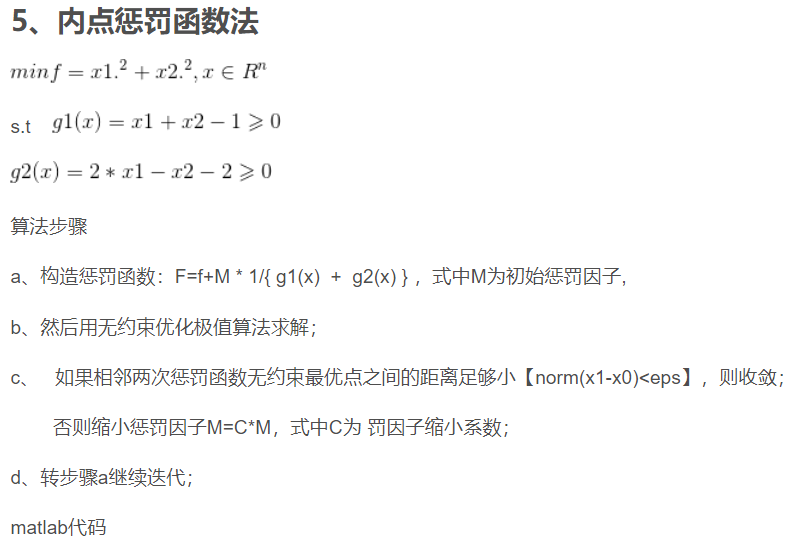%% 内点惩罚函数
syms x1 x2 x
f=x1.^2+x2.^2;
g=[x1+x2-1;2*x1-x2-2];
x0=[3 1];
M=10;
C=0.5;
eps=1e-6;
[x,result]=neidian(f,g,x0,M,C,eps,100)

function [x,result]=neidian(f,g,x0,M,C,eps,k)
% f 目标函数
% g 不等式约束函数矩阵
% h 等式约束函数矩阵
% x0 初始值
% M 初始障碍因子
% C 障碍因子缩小倍数
% eps 退出容差
% k 循环次数

%惩罚项
Neq=sum((1./g));

n=1;
while n<k
F=matlabFunction(f+M*Neq);
[x1,result]=Min_Newton(F,x0,eps,100);
x1=reshape(x1,1,length(x0));
tol=double(subs(Neq,symvar(Neq),x1)*M);
if tol < eps
if norm(x1-x0) < eps
x=x1;
result=double(subs(f,symvar(f),x));
break;
else
x0=x1;
M=M*C;
end
else
if norm(x1-x0) < eps
x=x1;
result=double(subs(f,symvar(f),x));
break;
else
x0=x1;
M=M*C;
end
end
n=n+1;
end
end

四、备注
完整代码或者代写添加QQ2449341593
往期回顾>>>>>>
【优化求解】基于matlab粒子群优化灰狼算法【含Matlab源码 006期】
【优化求解】基于matlab多目标灰狼优化算法MOGWO 【含Matlab源码 007期】
【优化求解】基于matlab粒子群算法的充电站最优布局【含Matlab源码 012期】
【优化求解】基于matlab遗传算法的多旅行商问题【含Matlab源码 016期】
【优化求解】基于matlab遗传算法求最短路径【含Matlab源码 023期】
【优化求解】基于matlab遗传和模拟退火的三维装箱问题【含Matlab源码 031期】
【优化求解】基于matlab遗传算法求解车辆发车间隔优化问题【含Matlab源码 132期】
【优化求解】磷虾群算法【含matlab源码 133期】
【优化求解】差分进化算法【含Matlab源码 134期】


展开全文• ConstrainedMPC.m 滚动求解，生成最优控制量。 ... function [k,r,y1,u1,x1,x2,x3,yout] = ConstrainedMPC(Ad,Bd,Cd,Nc,Np,rw,r,u_min,u_max,...%UNTITLED 此处显示有关此函数的摘要 % 此处显示详细说明 [Phi_Phi,Phi_F
ConstrainedMPC.m 滚动求解，生成最优控制量。

理论部分见https://blog.csdn.net/m0_37764065/article/details/107207726

function [k,r,y1,u1,x1,x2,x3,yout] = ConstrainedMPC(Ad,Bd,Cd,Nc,Np,rw,r,u_min,u_max,deltau_min,deltau_max)
%UNTITLED 此处显示有关此函数的摘要
%   此处显示详细说明
[Phi_Phi,Phi_F,PhiR,A_e,B_e,C_e]=mpcgain(Ad,Bd,Cd,Nc,Np);
% pole=[0.8 0.8 0.8 0.8];
% Kob=acker(A_e',C_e', pole)';
pole=[0.5 0.6 0.7 0.8];
Kob=zeros(size(A_e,1),1);
Kob=acker(A_e',C_e', pole)';
%Kob=[38.5114   32.6463   -9.9179    1.2720]';
[n,n_in]=size(B_e);
nd=size(Ad,1);
xm=zeros(nd,1);
Xf=zeros(n,1);
u=0;
y=0;
H=Phi_Phi+rw*eye(Nc);
Xr=zeros(n,1);
Xr(n,1)=r(1);
L0=zeros(Nc,1);
L0(1,1)=1;
A_cons=[L0';-L0';L0';-L0'];
b_d=[deltau_max;-deltau_min];
%A_cons_d=zeros(2*Nc,Nc);
%A_cons_a=zeros(2*Nc,Nc);
%for i=1:Nc
%    A_cons_d(i*2-1,i)=1;
%    A_consy_d(i*2,i)=-1;
%end
%for i=1:Nc
%    for j=1:i
%        A_cons_a(i*2-1,j)=1;
%        A_cons_a(i*2,j)=-1;
%    end
%end
%A_cons=[A_cons_d;A_cons_a];
%b_d=zeros(2*Nc,n_in);
%for i=1:Nc
%    b_d(i*2-1,:)=deltau_max;
%    b_d(i*2,:)=-deltau_min;
%end
%b_a=zeros(2*Nc,n_in);
N_sim=size(r,2);
for kk=1:N_sim
%for i=1:Nc
%b_a(i*2-1,:)=u_max-u;
%b_d(i*2,:)=-u_min+u;
%end
b_a=[u_max-u;-u_min+u];
b=[b_d;b_a];
Xr(n,1)=r(kk);
f=Phi_F*(Xf-Xr);%Phi_F*r(kk)=PhiR
DeltaU=QPhild(H,f,A_cons,b);
deltau=DeltaU(1,1);
Xf=A_e*Xf+B_e*deltau+ Kob*(y-C_e*Xf);
u=u+deltau;
x1(kk)=Xf(1,:);
x2(kk)=Xf(2,:);
x3(kk)=Xf(3,:);
yout(kk)=Xf(4,:);
u1(kk)=u;
y1(kk)=y;
xm_old=xm;
xm=Ad*xm+Bd*u;
y=Cd*xm;
%Xf=[xm-xm_old;y];
end
k=0:(N_sim-1);
end




展开全文• 一、简介 1 粒子群算法的概念 ...目前已被广泛应用于函数优化、神经网络训练、模糊系统控制以及其他遗传算法的应用领域。 2 粒子群算法分析 2.1基本思想 粒子群算法通过设计一种无质量的粒子来模拟鸟群中的
一、简介
1 粒子群算法的概念
粒子群优化算法(PSO：Particle swarm optimization) 是一种进化计算技术（evolutionary computation）。源于对鸟群捕食的行为研究。粒子群优化算法的基本思想：是通过群体中个体之间的协作和信息共享来寻找最优解．
PSO的优势：在于简单容易实现并且没有许多参数的调节。目前已被广泛应用于函数优化、神经网络训练、模糊系统控制以及其他遗传算法的应用领域。
2 粒子群算法分析
2.1基本思想
粒子群算法通过设计一种无质量的粒子来模拟鸟群中的鸟，粒子仅具有两个属性：速度和位置，速度代表移动的快慢，位置代表移动的方向。每个粒子在搜索空间中单独的搜寻最优解，并将其记为当前个体极值，并将个体极值与整个粒子群里的其他粒子共享，找到最优的那个个体极值作为整个粒子群的当前全局最优解，粒子群中的所有粒子根据自己找到的当前个体极值和整个粒子群共享的当前全局最优解来调整自己的速度和位置。下面的动图很形象地展示了PSO算法的过程：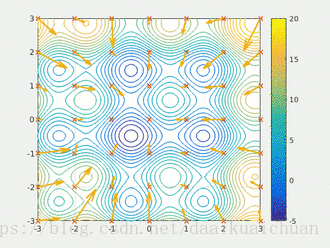2 更新规则
PSO初始化为一群随机粒子(随机解)。然后通过迭代找到最优解。在每一次的迭代中，粒子通过跟踪两个“极值”(pbest，gbest)来更新自己。在找到这两个最优值后，粒子通过下面的公式来更新自己的速度和位置。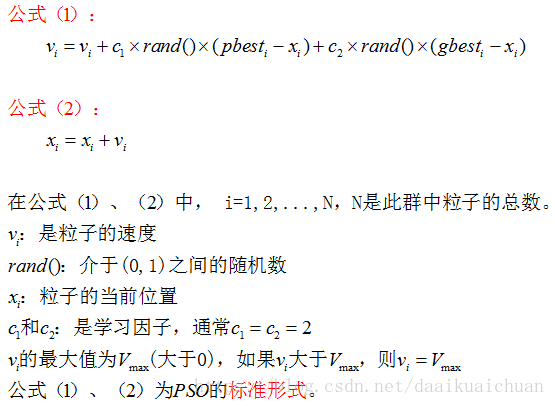公式(1)的第一部分称为【记忆项】，表示上次速度大小和方向的影响；公式(1)的第二部分称为【自身认知项】，是从当前点指向粒子自身最好点的一个矢量，表示粒子的动作来源于自己经验的部分；公式(1)的第三部分称为【群体认知项】，是一个从当前点指向种群最好点的矢量，反映了粒子间的协同合作和知识共享。粒子就是通过自己的经验和同伴中最好的经验来决定下一步的运动。以上面两个公式为基础，形成了PSO的标准形式。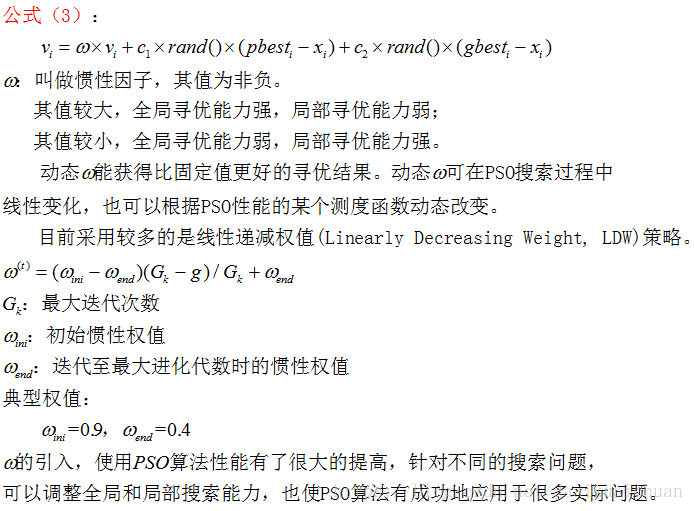公式(2)和 公式(3)被视为标准PSO算法。
3 PSO算法的流程和伪代码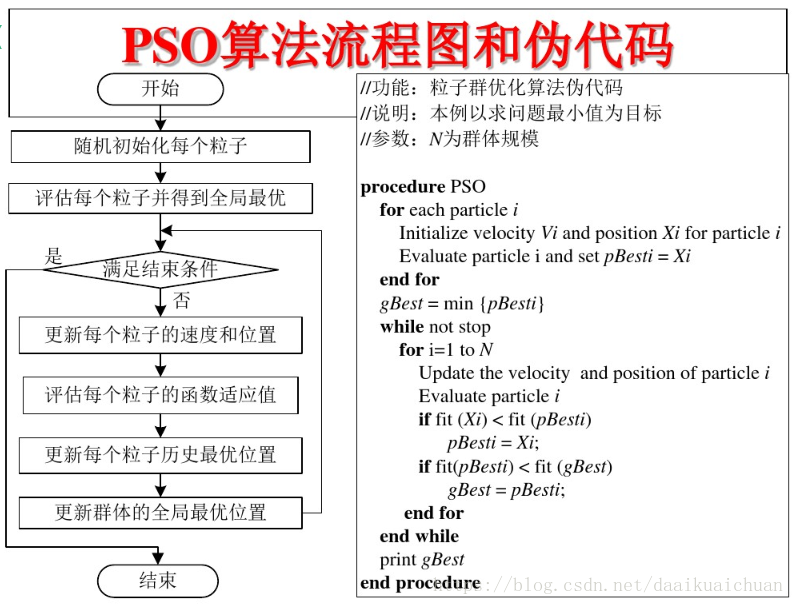二、源代码
clear all; clc; close all
%% 基础数据
%充电需求点坐标
b =1.0e+003.*[0.1092    0.1348
0.1197    0.2399
0.2578    0.1724
0.4259    0.1739
0.1257    0.3420
0.2803    0.3375
0.4439    0.3360
0.5505    0.1108
0.5610    0.2024
0.5700    0.3075
0.1332    0.4591
0.3013    0.4455
0.4559    0.4380
0.5850    0.4260
0.1452    0.6092
0.3163    0.5341
0.4739    0.5341
0.5880    0.5341
0.3193    0.6407
0.4784    0.6452
0.6015    0.6467
0.6736    0.1574
0.8657    0.1649
1.0308    0.1634
0.6781    0.2924
0.8327    0.2909
1.0188    0.2939
0.6811    0.4425
0.8192    0.4576
1.0083    0.4546
0.6691    0.5431
0.6976    0.6392
0.8191    0.6377
1.0098    0.6347];
%充电需求点常规电力负荷点负荷b(:,3)（kW）
b(:,3)=[2480;2480;8680;11400;890;2340;4160;560;1670;5010;2670;8280;7400;1430;7500;4840;3400;4290;3840;3680;2560;7000;14800;8960;3160;7000;5000;2280;10360;10000;760;6000;7040;5600];

%集中充电站坐标
bcs=[ 937.7296  379.5010
310.3141  238.4076];

na=4500;

alp=0.1;
b(:,4)=round(alp.*b(:,3)./sum(b(:,3)).*na);
b(23,4)=37;

ns=4;

mui=0.6;
Nchz=round(mui.*sum(b(:,4))./ns);

bm=1.0e+003*[0.0086,0.0088;1.1734,0.0088;1.1734,0.7412;0.0086,0.7412;0.0086,0.0088];

BL=sqrt(8.2*1.0e6./((max(bm(:,1))-min(bm(:,1)))*(max(bm(:,2))-min(bm(:,2)))));

Area2=1.0e+003 *[0.0086    0.0088
0.9377   -1.0860
0.3103    1.7040
0.0086    0.7412
0.0086    0.0088];
Area2=[Area2,2.*ones(size(Area2,1),1)];

Area1=1.0e+003 *[0.9377   -1.0860
1.1734    0.0088
1.1734    0.7412
0.3103    1.7040
0.9377   -1.0860];
Area1=[Area1,1.*ones(size(Area2,1),1)];

vv=[Area1;Area2];
for k=1:size(bcs,1)
Ai=find(vv(:,3)==k);
xx=vv(Ai,1);
yy=vv(Ai,2);
kk=convhull(xx,yy);
in=inpolygon(b(:,1),b(:,2),xx(kk),yy(kk));
b(in,5)=k;
end

Ep=[];

for i=1:size(bcs,1)
gb=b(b(:,5)==i,:);
Ep=[Ep;[sum(gb(:,4)),round(mui.*sum(gb(:,4))./ns),i]];
end

Tn=7;
PopSize=20;
MaxIter=300;
c1s=2.5;   c2s=0.5;
c1e=0.5;   c2e=2.5;
w_start=0.9;
w_end=0.4;
Iter=1;

xmax=max(Area1(:,1)); xmin=min(Area1(:,1));
ymax=max(Area1(:,2)); ymin=min(Area1(:,2));
x = xmin + (xmax-xmin).*rand(Tn,PopSize);
y = ymin + (ymax-ymin).*rand(Tn,PopSize);

X=[x;y];
V=rand(Tn*2,PopSize);
Vmax=0.1*max((xmax-xmin),(ymax-ymin));

inAr1=find(b(:,5)==1);
bb=[b(inAr1,1:2),b(inAr1,4)];
for pk=1:1:PopSize
[FX(pk),~,~,~,~,~,~,~,~,~]=VorCostCDEV(X(1:Tn,pk),X(Tn+1:end,pk),bb,bcs(1,:),BL);
end

PBest=X;
FPBest=FX;

[Fgbest,r]=min(FX);
Fm(Iter)=Fgbest;
CF=Fgbest;
Best=X(:,r);
FBest(Iter)=Fgbest;

FgNum=0;

while (Iter<=MaxIter)
Iter=Iter+1

w_now=((w_start-w_end)*(MaxIter-Iter)/MaxIter)+w_end;

A=repmat(X(:,r),1,PopSize);

R1=rand(Tn*2,PopSize);
R2=rand(Tn*2,PopSize);

c1=c1e+(c1s-c1e)*(1-acos(-2*Iter/(MaxIter+1)+1)/pi);
c2=c2e+(c2s-c2e)*(1-acos(-2*Iter/(MaxIter+1)+1)/pi);

V=w_now*V+c1*R1.*(PBest-X)+c2*R2.*(A-X);

changeRows=V>Vmax;
V(changeRows)=Vmax;

changeRows=V<-Vmax;
V(changeRows)=-Vmax;

X=X+1.0*V;

for pk=1:1:PopSize
[FX(pk),~,~,~,~,~,~,~,~,~]=VorCostCDEV(X(1:Tn,pk),X(Tn+1:end,pk),bb,bcs(1,:),BL);
end

P=FX<FPBest;
FPBest(P)=FX(P);
PBest(:,P)=X(:,P);

[Fgbest,r]=min(FPBest);
Fm(Iter)=Fgbest;

if Fgbest<CF
[FBest,gr]=min(FPBest);
Best=PBest(:,gr);
CF=Fgbest;
FgNum=0;
else
FgNum=FgNum+1;
end

if FgNum>10
SubX=r;
while SubX==r || SubX==0
SubX=round(rand*(PopSize));
end
r=SubX;
FgNum=0;
end

end
FBest
Best
Fm'

x =Best(1:Tn);
y =Best(Tn+1:end);
[Fcost,CCS,fcs,ucs,NchI,Epc,CVT,CVTI,CDL,CDLI]=VorCostCDEV(x,y,bb,bcs(1,:),BL)

%%%%%%%%%%%%%%%%%%%%%%%%%%%%%%%%%%%%%%%%%%%%%%%%%%%%%%%%%%%%%%%%%%%%%%%%%%
%% 作图
figure(1)

a=imread('T3.bmp');
imshow(a);
hold on

[vxT,vyT]=VoronoiT(bcs(:,1),bcs(:,2),0);
plot(bcs(:,1),bcs(:,2),'ks','linewidth',12);
plot(vxT,vyT,'k-','linewidth',3);

plot(b(:,1),b(:,2),'k*','linewidth',5)
plot(bm(:,1),bm(:,2),'k-','linewidth',3)

for k=1:length(b(:,4))
str=num2str(b(k,4));
text(b(k,1)-15,b(k,2)+25,str,'FontSize',23,'color','black');
end

for k=1:size(bcs,1)
str=num2str(k);
text(bcs(k,1)+20,bcs(k,2),str,'FontSize',20,'color','red');
end

三、运行结果四、备注
完整代码或者代写添加QQ 1564658423


展开全文• 一、简介 1 粒子群算法的概念 ...目前已被广泛应用于函数优化、神经网络训练、模糊系统控制以及其他遗传算法的应用领域。 2 粒子群算法分析 2.1基本思想 粒子群算法通过设计一种无质量的粒子来模拟鸟群中的
一、简介
1 粒子群算法的概念
粒子群优化算法(PSO：Particle swarm optimization) 是一种进化计算技术（evolutionary computation）。源于对鸟群捕食的行为研究。粒子群优化算法的基本思想：是通过群体中个体之间的协作和信息共享来寻找最优解．
PSO的优势：在于简单容易实现并且没有许多参数的调节。目前已被广泛应用于函数优化、神经网络训练、模糊系统控制以及其他遗传算法的应用领域。
2 粒子群算法分析
2.1基本思想
粒子群算法通过设计一种无质量的粒子来模拟鸟群中的鸟，粒子仅具有两个属性：速度和位置，速度代表移动的快慢，位置代表移动的方向。每个粒子在搜索空间中单独的搜寻最优解，并将其记为当前个体极值，并将个体极值与整个粒子群里的其他粒子共享，找到最优的那个个体极值作为整个粒子群的当前全局最优解，粒子群中的所有粒子根据自己找到的当前个体极值和整个粒子群共享的当前全局最优解来调整自己的速度和位置。下面的动图很形象地展示了PSO算法的过程：2 更新规则
PSO初始化为一群随机粒子(随机解)。然后通过迭代找到最优解。在每一次的迭代中，粒子通过跟踪两个“极值”(pbest，gbest)来更新自己。在找到这两个最优值后，粒子通过下面的公式来更新自己的速度和位置。公式(1)的第一部分称为【记忆项】，表示上次速度大小和方向的影响；公式(1)的第二部分称为【自身认知项】，是从当前点指向粒子自身最好点的一个矢量，表示粒子的动作来源于自己经验的部分；公式(1)的第三部分称为【群体认知项】，是一个从当前点指向种群最好点的矢量，反映了粒子间的协同合作和知识共享。粒子就是通过自己的经验和同伴中最好的经验来决定下一步的运动。以上面两个公式为基础，形成了PSO的标准形式。公式(2)和 公式(3)被视为标准PSO算法。
3 PSO算法的流程和伪代码二、源代码
%------主函数源程序－周调度（main.m）
%------基本粒子群优化算法（Particle Swarm Optimization）-----------
%------名称：基本粒子群优化算法（PSO）
%------作用：求解优化问题(周调度)
%------说明：全局性，并行性，高效的群体智能算法

%------初始格式化--------------------------------------------------
tic
clear all;
clc;
format short;
%% ------给定水电站初始化条件----------------------------------------------
%------------水电站1---------------------------------------
Vmax1=9015*10^4;        %水库容量上限(m3)
Vmin1=7000*10^4;         %水库容量下限(m3)
H1=640;                  %水库容量初始值水位(m)
V1=(2.0554*H1^2-2413.5002*H1+709934.65)*10^4 ;        %水库库容与水位的关系
h1=91;                   %初始水库水头(m)
qr(:,1:7)=[20.4 25.2 22.1 19.3 16.4 23.3 28.6 ];       %水库来水流量(m3/s)
qmax1=44;               %水库引用流量上限(m3/s)
qmin1=0;                 %水库引用流量下限(m3/s)
A1=9.8*10^3;             %水库出力系数
k1=0.65;                  %发电效率
t=8.64*10^4;            %水库发电引用流量时间段(s)
%-----------水电站2--------------------------------------
Vmax2=2080*10^4;        %水库容量上限(m3)
Vmin2=1530*10^4;         %水库容量下限(m3)
H2=540;                  %水库容量初始值水位(m)
V2=(2.6408*H2^2-2763.6392*H2+724014.90)*10^4;         %水库库容与水位的关系
h2=57;                   %初始水库水头(m)
qr(:,8:14)=[22.4 18.3 26.4 25.2 17.6 24.6 27.2];       %水库来水流量(m3/s)
qmax2=32;               %水库引用流量上限(m3/s)
qmin2=0;                 %水库引用流量下限(m3/s)
A2=9.8*10^3;             %水库出力系数
k2=0.6;                  %发电效率
%% 给定粒子群计算初始化条件
T=7;                   %周期
c1=2;                   %学习因子1
c2=2;                   %学习因子2
w1=0.9;                 %惯性权重
w2=0.4;
MaxIter=100;            %最大迭代次数
Iter=0;
vmax=2;                %最大运动速度
vmin=0;               %最小运动速度
N=20;                   %初始化群体个体数目
%------初始化种群的个体(可以在这里限定位置和速度的范围)------------

q=zeros(N,2*T);      %位置随机初始化位置
v=rand(N,2*T);           %速度随机初始化速度

%------适应度函数源程序（fitness.m）----------------------------

Fx1=sum((k1*A1*q(:,1:7).*h1*t/(3.6*10^10))')';    %水库1总发电量（万kWh）
Fx2=sum((k2*A2*q(:,8:14).*h2*t/(3.6*10^10))')';    %水库2总发电量（万kWh）
Fx=Fx1+Fx2;   %水库总发电量（万kWh）

%------设置当前粒子的最好位置----------------------------------

Pbest=q;
FPbest=Fx;
%------找到初始粒子的最好粒子------------------------------------
[Fgbest,r]=max(Fx);
E=Fgbest;%记录当前全局最优值
Best=q(r,:);%用于保存最优粒子位置

%% ------------------------------循环-----------------------------

while(Iter<=MaxIter)
Iter=Iter+1;

%------------------------------更新惯性权重和速度----------------------

w=((w1-w2)*(MaxIter-Iter)/MaxIter)+w2;% 典型的线性递减策略
% 线性微分递减策略
% 先增后减策略
% 非线性惯性权重策略
R1=rand(N,2*T);
R2=rand(N,2*T);

%-------------------------粒子速度更新----------------------------

B=repmat(q(r,:),N,1);
v=w*v+c1*R1.*(Pbest-q)+c2*R2.*(B-q);

%-------------------------粒子速度约束----------------------------

changev=v>vmax;
v(find(changev))=vmax;
changev=v<vmin;
v(find(changev))=vmin;

%-------------------------粒子流量更新----------------------------

q=q+1.0*v;

%-------------------------各时段引用流量约束----------------------------
for i=1:7
q1=q(:,i);
changeq1=q1>qmax1;
q1(find(changeq1))=qmax1;
changeq1=q1<qmin1;
q1(find(changeq1))=qmin1;
q(:,i)=q1;
end
for i=8:14
q2=q(:,i);
changeq2=q2>qmax1;
q2(find(changeq2))=qmax1;
changeq2=q2<qmin1;
q2(find(changeq2))=qmin1;
q(:,i)=q2;
end

%-------------------------各时段库容大小(m3)----------------------------
qr1=qr(:,1:7);
qr2=qr(:,8:14);
Qr1=repmat(qr1,N,1);        %----各时段来水大小
Vs1=repmat(0,N,T+1);       %----各时段初始库容大小
Vj1=repmat(0,N,T);         %----各时段库容大小(首末平均值)
quit1=repmat(0,N,T);       %----各时段弃水大小

Qr2=repmat(qr2,N,1);        %----各时段来水大小
Vs2=repmat(0,N,T+1);       %----各时段初始库容大小
Vj2=repmat(0,N,T);         %----各时段库容大小(首末平均值)
quit2=repmat(0,N,T);       %----各时段弃水大小

%-------------------------各时段水头大小----------------------------------

for i=1:N
Vs1(i,1)=V1;
Vs2(i,1)=V2;
end

for i=1:N
for j=2:(T+1)
q1=q(:,1:7);
Vs1(i,j)=Vs1(i,(j-1))+(Qr1(i,(j-1))-q1(i,(j-1)))*t;

if Vs1(i,j)>=Vmax1
quit1(i,j-1)= Vs1(i,j)-Vmax1;
Vs1(i,j)=Vmax1;
end
if Vs1(i,j)<=Vmin1
q1(i,j-1)=(Vs1(i,j)-Vmin1+Qr1(i,j-1)*t)/(t);
Vs1(i,j)=Vmin1;
end
end
end

for i=1:N
for j=2:(T+1)
q2=q(:,8:14);
Vs2(i,j)=Vs2(i,(j-1))+(Qr2(i,(j-1))-q2(i,(j-1)))*t+quit1(i,(j-1))+q1(i,j-1)*t;
if Vs2(i,j)>=Vmax2
quit2(i,j-1)= Vs2(i,j)-Vmax2;
Vs2(i,j)=Vmax2;
end
if Vs2(i,j)<=Vmin2
q2(i,j-1)=(Vs2(i,j)-Vmin2+Qr2(i,j-1)*t)/(t);
Vs2(i,j)=Vmin2;
end
end
end

for i=1:N
for j=1:T
Vj1(i,j)=(Vs1(i,j)+Vs1(i,j+1))/2;
Vj2(i,j)=(Vs2(i,j)+Vs2(i,j+1))/2;
end
end

三、运行结果四、备注
完整代码或者代写添加QQ 1564658423


展开全文• 一、简介 1 粒子群算法的概念 ...目前已被广泛应用于函数优化、神经网络训练、模糊系统控制以及其他遗传算法的应用领域。 2 粒子群算法分析 2.1基本思想 粒子群算法通过设计一种无质量的粒子来模拟鸟群中的
• 受粒子群优化算法的启发,提出一种控制参数a随机动态调整策略.此外,为了提高算法的全局收敛速度,用混沌初始化方法产生初始种群.采用18个高维测试函数进行仿真实验,结果表明:对于绝大多数情形,在相同最大适应度函数...
• 图像增强是一种必要的...蜻蜓算法(DA)直观的优化技术,这是首选工程应用中,已被用于优化提出了传递函数参数值。 % Using the Regional Similarity Transformation Function and Dragonfly Algorithm. %%%%%%%%%%
• 零件的参数设计的模型分析 摘要 本文以产品的成本和产品期望损失之和为 目标函数以标定值和容差 为变量建立非线性优化模型 问题中产品的参数 7 个零件参数确定零件参数 标定值和容差共 同确定零件的容差越小产品参数...
• 基于径向基核函数的最小二乘支持向量机参数主要涉及惩罚因子和核函数参数，这两个参数的选择将直接影响最小二乘支持向量机的学习和泛化能力。为了提高最小二乘支持向量机的预测结果，文章用灰狼优化算法对其参数寻优...
• 一、简介 1 粒子群算法的概念 ...目前已被广泛应用于函数优化、神经网络训练、模糊系统控制以及其他遗传算法的应用领域。 2 粒子群算法分析 2.1基本思想 粒子群算法通过设计一种无质量的粒子来模拟鸟群中的
• 一、简介 1 粒子群算法的概念 ...目前已被广泛应用于函数优化、神经网络训练、模糊系统控制以及其他遗传算法的应用领域。 2 粒子群算法分析 2.1基本思想 粒子群算法通过设计一种无质量的粒子来模拟鸟群中的
• 3、这样我们就得到了N组并且每组解的个数为D的集合，它可以使用N个D维参数向量来表示。 因为它类似于遗传算法进化一样，是一代一代的进行进化，最终得到最优个体。所以上面G表示的就是代数。 形象表示如下： 二、...
• 现有的LSSVM工具箱，自带PSO优化参数无需调整,Matlab编写的人工蜂群算法代码,详细注释和测试函数,简短易懂,执行顺畅。可用于解决无约束优化问题。LSSVM
• 基于DE的EA，具有自适应交叉率，人口刷新和本地搜索。 该工具集包含以下文件： 1）DE_TCR.m 运行优化算法。该代码实现了DE_TCR算法的版本 ...2）RunTutorial.m ...要优化的成本函数。 获取代码：htt...
• 由于它仅依靠适应度函数就可以搜索最优解，不需要有关问题解空间的知识，并且适应度函数不受连续可微等条件的约束，因此在解决多维、高度非线性的复杂优化问题中得到了广泛应用和深入研究。 遗传算法在模式识别、...
• 一、简介 1 粒子群算法的概念 ...目前已被广泛应用于函数优化、神经网络训练、模糊系统控制以及其他遗传算法的应用领域。 2 粒子群算法分析 2.1基本思想 粒子群算法通过设计一种无质量的粒子来模拟鸟群中的
• 一、简介 1 粒子群算法的概念 ...目前已被广泛应用于函数优化、神经网络训练、模糊系统控制以及其他遗传算法的应用领域。 2 粒子群算法分析 2.1基本思想 粒子群算法通过设计一种无质量的粒子来模拟鸟群中的
• 现有的LSSVM工具箱，自带PSO优化参数无需调整,Matlab编写的人工蜂群算法代码,详细注释和测试函数,简短易懂,执行顺畅。可用于解决无约束优化问题。
• 控制量变化权重rw越小闭环响应越快，越大说明控制量变化在优化函数占权重越大。 目录 1.建模 2.离散化 3.建立增广矩阵 4.预测 5.优化 5.1 定义二次型优化函数 5.2 变量约束 5.3 优化求解 6.滚动 7....
• 一、简介 1 粒子群算法的概念 ...目前已被广泛应用于函数优化、神经网络训练、模糊系统控制以及其他遗传算法的应用领域。 2 粒子群算法分析 2.1基本思想 粒子群算法通过设计一种无质量的粒子来模拟鸟群
• - 在 Simulink 中提供可调参数结构丶触发模型块以及用于大型建模的函数调用分支 - 在 嵌入式 IDE 链接和目标支持包中提供针对 Eclipse丶嵌入式 Linux 及 ARM 处理器的代码生成支持 - 在 IEC 认证工具包中提供对 ...
• 为了提高SVM数据的分类预测准确率，对SVM中惩罚参数和核函数参数利用麻雀搜索算法进行优化。 1.数据集 wine 数据的来源是 UCI 数据库 ， 记录的是在意大利同一区域里三种不同品种的葡萄酒的化学成分分析，数据里含有...
• 该书共有30个MATLAB神经网络的案例(可运行程序)，包括BP、RBF、SVM、SOM、Hopfield、LVQ、Elman、小波等神经网络;还包含PSO(粒子群)、灰色神经网络、模糊网络、概率神经网络、遗传算法优化等内容。该书另有31个...
• , 该书共有30个MATLAB神经网络的案例(可运行程序），包括BP、RBF、SVM、SOM、Hopfield、LVQ、Elman、小波等神经网络；还包含PSO(粒子群）、灰色神经网络、模糊网络、概率神经网络、遗传算法优化等内容。该书另有31...
• 该书共有30个MATLAB神经网络的案例(可运行程序），包括BP、RBF、SVM、SOM、Hopfield、LVQ、Elman、小波等神经网络；还包含PSO(粒子群）、灰色神经网络、模糊网络、概率神经网络、遗传算法优化等内容。该书另有31个...
• 10．1．1 优化工具箱常用函数 10．1．2 最优化问题 10．2 线性规划 10．2．1 线性规划函数 10．2．2 线性规划问题的应用 10．3 约束非线性规划 10．3．1 基本数学原理介绍 10．3．2 约束非线性规划函数 10．3．3 ...
• 10．1．1 优化工具箱常用函数 10．1．2 最优化问题 10．2 线性规划 10．2．1 线性规划函数 10．2．2 线性规划问题的应用 10．3 约束非线性规划 10．3．1 基本数学原理介绍 10．3．2 约束非线性规划函数 10．3．3 ...
• 区间优化工具以及程序，INTLAB完全是用Matlab编写的.自我验证方法 密集的线性系统（也包括内部包含和结构化矩阵） 稀疏的spd线性系统 非线性方程组 几个全球性例程： 非线性方程组的所有根 验证了全局优化 验证约束...# matlab含参数函数优化matlab 订阅